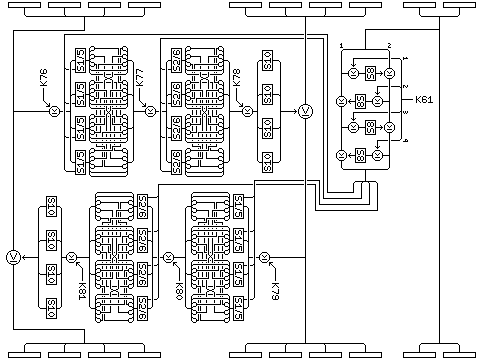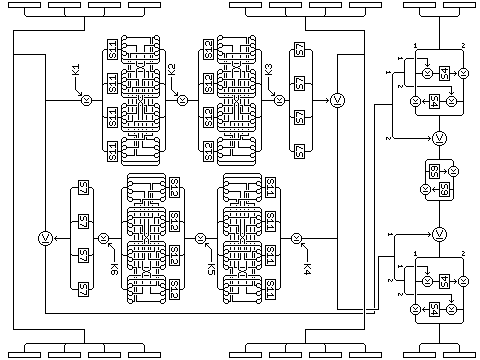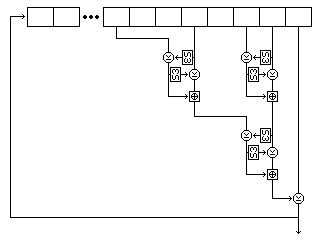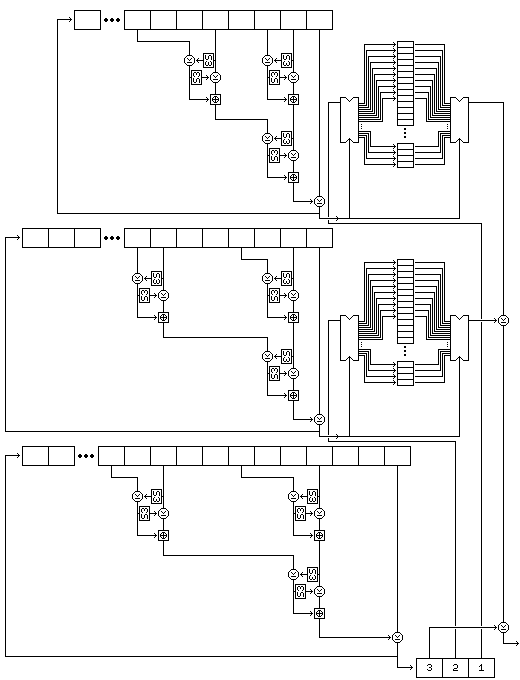[Next] [Up] [Previous] [Index]

Quadibloc 2002C is a block cipher with a block size of 80 bits. This unusual block size makes it simple to devise a cipher illustrating the particular type of unbalanced Feistel round which serves as the underlying principle of this cipher.

There are two types of round in Quadibloc 2002C. The first type of round, which shall be referred to as the Type A round, uses an f-function of 16 bits of the block to control two Feistel rounds using the standard Quadibloc f-function which modify the other 64 bits of the block, and is as illustrated below:The second type of round uses two Feistel rounds with the standard Quadibloc f-function as an f-function in itself, by means of which 64 bits of the block produce a 64 bit value used to modify the other 16 bits of the block. This round, the type B round, is illustrated here:The type B round is felt to contribute to the strength of the cipher because it shares with some of the better stream ciphers the characteristic that the modification of the block which it produces, while it contains only 16 bits of information, depends on 64 bits of input, and on 192 bits of subkey, thus making it difficult to work backwards to make deductions concerning the subkeys. However, the fact that the effects of the subkeys are confined to 16 bits of the block means that this round produces slow diffusion by itself, and thus it is complemented by the type A round.

### The Cipher in Detail

The encipherment of an 80-bit block of plaintext by means of Quadibloc 2002C shall consist of the following steps:

• Five type A rounds, each one followed by a plain byte permutation.
• Five sequences of one type A round, followed by one type B round, followed by a twisted byte permutation.
• Five type A rounds, with a plain byte permutation between each pair of them, for a total of four byte permutations.
• Five sequences of a twisted byte permutation, followed by a type B round, followed by a type A round.
• Five type A rounds, each one preceded by a plain byte permutation.

A plain byte permutation takes the ten bytes in a block from the order:

```  1  2  3  4  5  6  7  8  9 10
```

to the order:

```  3  4  9  1  8 10  5  6  2  7
```

and a twisted byte permutation takes the ten bytes in a block from the order:

```  1  2  3  4  5  6  7  8  9 10
```

to the order:

``` 10  1  2  3  9  5  6  7  4  8
```

thus, the plain byte permutations are used between type A rounds so as to preserve alternation between the left and right halves of the Feistel round, while the twisted byte permutation is used when type B rounds are also present, since in that case, the two additional bytes are modified together, and thus they can be swapped between streams. Also note that the plain byte permutation rotates the two groups of five bytes by either two or three bytes per step, while the twisted byte permutation rotates a loop including all ten bytes by only one byte per step. Thus, the plain byte permutation brings different bytes of each half of the block into corresponding positions in succeeding rounds.

The type A round proceeds as follows:

The block is divided into ten bytes, numbered 0 through 9 from left to right.

• A copy of bytes 8 and 9 are enciphered by means of four miniature Feistel rounds as follows:
• A copy of byte 8 is XORed with the leftmost byte of the current subkey for this purpose (K61 for the first type A round, K62 for the next, and so on), the element of the key-dependent S-box S8 to which it is the index is taken, and that result is XORed with the copy of byte 9, modifying it.
• A copy of byte 9 is then XORed with the second leftmost byte of K61 or the successor thereof, it is used as an index into S8, and the result is XORed with the copy of byte 8, modifying it.
• Using the third and fourth bytes of K61 or its successor, two additional Feistel rounds following the pattern of those preceding are performed.
• The 16-bit result of these Feistel rounds is used, a bit at a time, to select S boxes for standard Quadibloc f-functions.
• A copy of bytes 0 to 3 is used as input into a standard Quadibloc f-function, the output of which is XORed with bytes 4 to 7, modifying them. The f-function proceeds as follows:
• Subkey K76 is XORed with the copy of bytes 0 to 3 in the first Type A round, subkey K82 used in the next, increasing by six with each round.
• The first four bits of the 16-bit result above select between S-boxes 1 and 5 as derived from Euler's Constant in the first subsitution/permutation layer of the f-function. The permutation layer is as described previously for other ciphers of the Quadibloc series.
• Subkey K77 or the successor thereof is XORed with the result.
• The next four bits of that result select between S-boxes 2 and 6 in the second substitution/permutation layer.
• Subkey K78 or the successor thereof is XORed with the result.
• The bytes of the result are replaced by their substitutes from key-dependent S-box S10.
• A copy of bytes 4 through 7 as modified is then used as input to a similar f-function, using the latter eight bits of the result of the small Feistel rounds applied to a copy of bytes 8 and 9, and subkeys K79, K80, and K81 in the first type A round or the successors thereof, and the result is XORed with bytes 0 through 3, modifying them.

The type B round proceeds as follows:

• A copy of bytes 0 through 7 is used as input to two Feistel rounds using the Quadibloc f-function. In the first round, bytes 0 through 3 are the input, bytes 4 through 7 are modified, the subkeys are K1, K2, and K3 in the first type B round, incremented by 6 between rounds, and the S-boxes are S11 and S12 as derived from Euler's constant in the two S/P layers, and the key-dependent S-box S7 at the end. In the second Feistel round within the first Type B round, subkeys K4, K5, and K6 are used, and the modified bytes 4 through 7 are used as input to produce a result modifying the copy of bytes 0 to 3.
• The 64-bit result is used to modify bytes 8 and 9, the only bytes of the block actually changed by a Type B round, as follows:
• A copy of byte 8 is XORed with the first byte of the eight-byte result, used as an index into S-box S4 as derived from Euler's constant, and the result is XORed with byte 9.
• A copy of byte 9 is XORed with the second byte of the eight-byte result, used as an index into S-box S4, and the result is XORed with byte 8.
• The third and fourth bytes are XORed with bytes 8 and 9 in a 16-bit XOR operation.
• A copy of byte 8 is used as an index into key-dependent S-box S9, and the result is XORed with byte 9.
• A copy of byte 9 is used as an index into key-dependent S-box S9, and the result is XORed with byte 8.
• The fifth and sixth bytes are XORed with bytes 8 and 9 in a 16-bit XOR operation.
• A copy of byte 8 is XORed with the seventh byte of the eight-byte result, used as an index into S-box S4, and the result is XORed with byte 9.
• A copy of byte 9 is XORed with the eighth byte of the eight-byte result, used as an index into S-box S4, and the result is XORed with byte 8.

### The Key Schedule

The subkey material used by Quadibloc 2002C is as follows:

• Sixty 32-bit subkeys, K1 through K60, used in the ten type B rounds, six to a round, for the two Feistel rounds which serve as the f-function by which 64 bits of the block modify the other 16 bits;
• Fifteen 32-bit subkeys, K61 through K75, used in the small Feistel rounds within the type A rounds, which serve as the f-function by which 16 bits of the block control the large Feistel rounds through which the other 64 bits of the block are modified;
• Ninety 32-bit subkeys, K76 through K165, used in the main Feistel rounds of the type A rounds, six to a round;
• Four bijective S-boxes containing a permutation of the values from 0 to 255, S7, S8, S9, and S10.

Subkey generation proceeds in the following order:

• K1 through K60 are generated.
• S7 is generated.
• A noninvertibility operation is performed.
• K61 through K75 are generated.
• S8 is generated.
• A noninvertibility operation is performed.
• S9 is generated.
• A noninvertibility operation is performed.
• K76 through K165 are generated.
• S10 is generated.

The purpose of the noninvertibility operation is to serve as a one-way function, like a hash function, so that even if information about K76 through K165, the subkeys used in the most conventional manner in Feistel rounds whose result is directly manifest in the relation between plaintext and ciphertext, can be obtained through methods such as differential cryptanalysis, this information will not lead to information about subkeys K1 through K60, which are in the portion of the cipher which is hidden away from analysis because it affects only 16 bits of the block at a time.

The key will be a multiple of four bytes in length, and must be at least eight bytes in length.

Once again, the methods used to generate subkey bytes and key-dependent permutations are as follows:

### Initialization

Three strings of bytes of different length are produced from the key.

The first string consists of the key, followed by one byte containing the one's complement of the XOR of all the bytes of the key.

The second string consists of the one's complements of the bytes of the key in reverse order, with three bytes appended containing the following three quantities:

• The sum, modulo 255, of the bytes of the key, incremented by one by normal addition. (Thus, this produces a number from 1 to 255.)
• The XOR of all the bytes at odd numbered positions in the key, where the first byte in the key is considered to be byte 1, and odd.
• The one's complement of the XOR of all the bytes at even numbered positions in the key.

The third string consists of alternating bytes, taken from the bytes of the key in reverse order, and then from the bytes of the one's complement of the key, and then that string is followed by the one's complements of the first four bytes of the key.

Thus, if the key is:

``` 128  64  32  16   8   4   2   1   1   2   3   4   5   6   7   8
```

then the strings generated from it are as follows:

```First string:
128  64  32  16   8   4   2   1
1   2   3   4   5   6   7   8
8

Second string:
247 248 249 250 251 252 253 254
254 253 251 247 239 223 191 127
37 170  93

Third string:
8 127   7 191   6 223   5 239
4 247   3 251   2 253   1 254
1 254   2 253   4 252   8 251
16 250  32 249  64 248 128 247
127 191 223 239
```

Given that the length of the key is 4n, the lengths of the three strings are 4n+1, 4n+3, and 8n+4, and hence all three are relatively prime, since both 4n+1 and 4n+3 are odd, and 8n+4 is two times 4n+2.

Two buffers, each containing room for 256 bytes, are filled by generating bytes from the first and second strings by placing them in a nonlinear shift register.

The form of that shift register is shown in the following illustration, showing its precise form for the first string.Five bytes are read from the string at each step. For the first string, they are, as shown in the diagram, the eighth-last, fifth-last, third-last, and second-last bytes and the last byte. For the second string, they are the eighth-last, seventh-last, fourth-last, and second-last bytes, and the last byte. For the third string, they are the twelfth-last, tenth-last, seventh-last, and fourth-last bytes, and the last byte.

Each time the shift register produces a byte, it does so as follows:

• The second byte read is used to select an entry in S-box S3, and the value of this entry is XORed to the first byte. Then the first byte is used to select an entry in S-box S3, and the value of this entry is XORed to the second byte.
• The fourth byte read is used to select an entry in S-box S3, and the value of this entry is XORed to the third byte. Then the third byte is used to select an entry in S-box S3, and the value of this entry is XORed to the fourth byte.
• The first and second bytes, as modified, are added together using modulo-256 addition to form a first result.
• The third and fourth bytes, as modified, are added together using modulo 256 addition to form a second result.
• The second result is used to select an entry in S-box S3, and the value of this entry is XORed to the first result. Then the first result is used to select an entry in S-box S3, and the value of this entry is XORed to the second result.
• The two results as modified are added together using modulo 256 addition to form a third result.
• The fifth byte read, which is always the last byte in the shift register, is XORed with the third result. The resulting value is output as the byte produced by operating the shift register.
• The values in the shift register are modified by removing the last byte, advancing the bytes in the shift register to the next later position, and appending the output result as the new first byte of the shift register contents.

Both buffers contain 256 bytes. The first buffer, called buffer A, is filled with 256 successive bytes generated from the second string by means of a nonlinear shift register filled with the second string. The second buffer, called buffer B, is filled with 256 successive bytes generated from the first string by means of the nonlinear shift register filled with the first string.

### Subkey Byte Generation

Once the setup is complete, subkey material is generated one byte at a time, the first byte generated being the leftmost byte of subkey K1, and so on.

A subkey byte is generated as follows:

• A byte is generated from the first string by the nonlinear shift register operation.
• The byte at the position in buffer A indicated by this value is taken, and is called P.
• A byte is generated from the third string by the nonlinear shift register operation. In the case of the third string, the shift register operation involves the following five bytes: the twelfth, tenth, seventh, and fourth last bytes, and t byte. The value of the byte thus produced is placed in buffer A, replacing the
• A byte is generated from the second string by the nonlinear shift register operation.
• The byte at the position in buffer B indicated by this value is taken, and Q.
• A byte is generated from the third string by the nonlinear shift register operation. Its value is placed in buffer B, replacing the value taken.
• The subkey byte generated is the XOR of P and Q and an additional byte generated from the third string by the nonlinear shift register operation. (The use of an additional byte from the third string ensures that all the bytes of the key participate directly in the key schedule; otherwise, some could be skipped over in a sense as a result of the selection of bytes to use from the buffers.)

The following diagram attempts to illustrate the complete process of subkey byte generation:Note that this procedure, since it exercises the two strings used to select bytes, rather than the string used to generate values, results in a small change in the key bringing about large changes in the subkeys from the very beginning, although it is still true, since the bytes used to select buffer contents could be the same by coincidence, that subkeys generated from very similar keys have a slightly enhanced probability of having bytes in common.

### Permutation Generation

During subkey generation, the key-dependent S-boxes S7, S8, S9 and S10 must be generated. To generate each bijective S-box, the following procedure is used:

• 256 bytes are generated following the same procedure as for subkey byte generation, and these bytes are placed in a 256-byte buffer called buffer C.
• A 256-byte buffer called buffer D is filled with the numbers from 0 to 255 in order.
• For every i from 0 to 255, if element i of buffer C (hereinafter called C(i)) is not equal to i, swap elements i and C(i) of buffer D.
• For every i from 0 to 255, if B(i) is not equal to i, swap elements i and B(i) of buffer D.
• For every i from 0 to 255, if A(i) is not equal to i, swap elements i and A(i) of buffer D.

The resulting contents of buffer D are used as the key-dependent bijective S-box intended to be produced. Note that this is a procedure, introduced for Quadibloc X, is more straightforwards than the other two basic procedures used previously to produce S8 in other ciphers in this series.

This procedure, although it uses buffers A and B, leaves them undisturbed; thus, byte generation may continue after one S-box is produced.

### The Noninvertibility Operation

The noninvertibility operation, a step in this plan of subkey generation introduced especially for Quadibloc 2002C, consists of generating as many bytes of subkey material as there are bytes in the three shift registers used the initial contents of which were derived from the keys, as well as an additional 512 bytes of subkey material, and then XORing this subkey material, in order, with the bytes of those three shift registers, the bytes of buffer A, and the bytes of buffer B.

### Long Keys

The subkey generation procedure given above works well for keys from 64 bits to 1,024 bits in length. When the length of the key approaches 2,048 bits in length, however, the first two shift registers are no longer thoroughly mixed by filling buffers initially.

Thus, the following modified subkey generation procedure is given for keys which are more than 1,024 bits long. A key must still be a multiple of 32 bits in length:

• (1) Divide the key into blocks of 512 bits until the remaining part of the key is from 512 to 992 bits in length.
• (2) Use the first block of the key as the key for the normal Quadibloc 2002C key schedule.
• (3) Continue to generate enough additional subkey bytes to XOR these with the block into which the key was divided.
• (4) Use the next block, after the XOR, to carry out the Quadibloc 2002C key schedule, except:
• The subkey material generated is XORed with the previously generated values of the subkeys;
• (This is different for Quadibloc 2002C) For the second, and all subsequent even-numbered, blocks, generate the values used to modify the existing keys in the reverse sequence of groups between noninvertibility operations, but in the normal order within each one (for odd-numbered blocks, use the initial order); that is:
• K76 through K165 are generated.
• S10 is generated.
• A noninvertibility operation is performed.
• S9 is generated.
• A noninvertibility operation is performed.
• K61 through K75 are generated.
• S8 is generated.
• A noninvertibility operation is performed.
• K1 through K60 are generated.
• S7 is generated.
• Generate permutations for key-dependent S-boxes S7 through S10 in the normal sequence of generation, and then modify the S8 and S9 to be used in the cipher as follows: go through the newly-generated S-box and the previously calculated corresponding S-box from positions 0 to 255; in a new array, place the value in the new permutation in the position indicated by the value in the original permutation. Once this is finished, the new array is the replacement permutation.
• (5) If there are any additional blocks remaining in the key, go back to ste

This method ensures that the key schedule is a function of the entire key. It also is expected to ensure that there is no usable relationship between the groups of keys separated by noninvertibility operations.

[Next] [Up] [Previous] [Index]